Download Presentation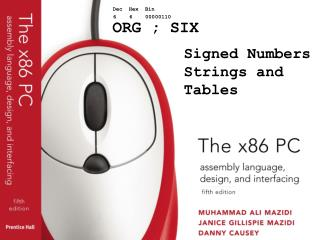ORG ; SIX

# ORG ; SIX - PowerPoint PPT Presentation

Dec Hex Bin. 6 6 00000110. ORG ; SIX. Signed Numbers Strings and Tables. OBJECTIVES this chapter enables the student to:. Convert a number to its 2’s complement. Code signed arithmetic instructions: ADD, SUB, IMUL, and IDIV.I am the owner, or an agent authorized to act on behalf of the owner, of the copyrighted work described.
Download Presentation## ORG ; SIX

An Image/Link below is provided (as is) to download presentation

Download Policy: Content on the Website is provided to you AS IS for your information and personal use and may not be sold / licensed / shared on other websites without getting consent from its author.While downloading, if for some reason you are not able to download a presentation, the publisher may have deleted the file from their server.

- - - - - - - - - - - - - - - - - - - - - - - - - - E N D - - - - - - - - - - - - - - - - - - - - - - - - - -
Presentation Transcript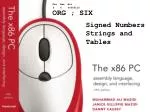Dec Hex Bin

6 6 00000110

ORG ; SIX

Signed Numbers Strings and Tables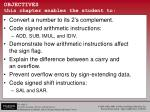OBJECTIVESthis chapter enables the student to:
• Convert a number to its 2’s complement.
• Code signed arithmetic instructions:
• ADD, SUB, IMUL, and IDIV.
• Demonstrate how arithmetic instructions affectthe sign flag.
• Explain the difference between a carry andan overflow.
• Prevent overflow errors by sign-extending data.
• Code signed shift instructions:
• SAL and SAR.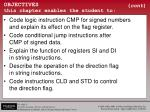OBJECTIVESthis chapter enables the student to:

(cont)

• Code logic instruction CMP for signed numbersand explain its effect on the flag register.
• Code conditional jump instructions afterCMP of signed data.
• Explain the function of registers SI and DIin string instructions.
• Describe the operation of the direction flagin string instructions.
• Code instructions CLD and STD to controlthe direction flag.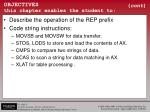OBJECTIVESthis chapter enables the student to:

(cont)

• Describe the operation of the REP prefix
• Code string instructions:
• MOVSB and MOVSW for data transfer.
• STOS, LODS to store and load the contents of AX.
• CMPS to compare two strings of data.
• SCAS to scan a string for data matching in AX.
• XLAT for table processing .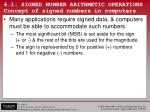6.1: SIGNED NUMBER ARITHMETIC OPERATIONS Concept of signed numbers in computers
• Many applications require signed data, & computers must be able to accommodate such numbers.
• The most significant bit (MSB) is set aside for the sign(+ or -) & the rest of the bits are used for the magnitude.
• The sign is represented by 0 for positive (+) numbersand 1 for negative (-) numbers.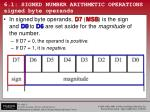• In signed byte operands, D7 (MSB) is the signand D0toD6 are set aside for the magnitude ofthe number.
• If D7 = 0, the operand is positive.
• If D7 = 1, it is negative.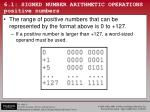6.1: SIGNED NUMBER ARITHMETIC OPERATIONS positive numbers
• The range of positive numbers that can be represented by the format above is 0 to +127.
• If a positive number is larger than +127, a word-sized operand must be used.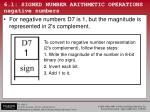To convert to negative number representation(2's complement), follow these steps:

1. Write the magnitude of the number in 8-bit binary (no sign).

2. Invert each bit.

3. Add 1 to it.

1

6.1: SIGNED NUMBER ARITHMETIC OPERATIONS negative numbers
• For negative numbers D7 is 1, but the magnitude is represented in 2's complement.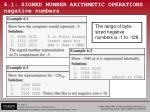6.1: SIGNED NUMBER ARITHMETIC OPERATIONS negative numbers

The range of byte-sized negative numbers is -1 to -128.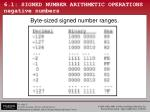• A word is 16 bits in length in x86 computers.
• Setting aside the MSB (D15) for the sign leavesa total of 15 bits (D14– D0) for the magnitude.
• A range of -32768 to +32767.
• Larger numbers is must be treated as a multiword operand, processed chunk by chunk.
• The same way as unsigned numbers.The range of signed word operands.2. Invert each bit.

3. Add 1 to it.

• To convert a negative number to its word operand representation, the same three steps discussed in negative byte operands are used:6.1: SIGNED NUMBER ARITHMETIC OPERATIONS signed number operations overflow problem
• When using signed numbers, a serious issue, the overflow problem, must be dealt with.
• The CPU understands only 0s & 1s, ignoring thehuman convention of positive/negative numbers.
• The CPU indicates the problem with the OF (overflow) flag.
• If the result of an operation on signed numbers is too large for the register, an overflow occurs.
• Review Example 6-4.The CPU designers created the overflow flagto inform the programmer that the result ofthe signed number operation is erroneous.

6.1: SIGNED NUMBER ARITHMETIC OPERATIONS signed number operations overflow problem

+96 is added to +70 and the result according to the CPU is -90.

Why? The result was more than AL could handle, because likeall other 8-bit registers, AL could only contain up to +127.6.1: SIGNED NUMBER ARITHMETIC OPERATIONS the overflow flag in 8-bit operations
• In 8-bit signed number operations, OF is set to 1 if:
• There is a carry from D6 to D7 but no carry out of D7.
• (CF = 0)
• There is a carry from D7 out, but no carry from D6 to D7.
• (CF = 1)
• The overflow flag is set to 1 if there is a carry from D6 to D7 or from D7 out, but not both.
• If there is a carry both from D6 to D7, and from D7 out,then OF = 0.6.1: SIGNED NUMBER ARITHMETIC OPERATIONS the overflow flag in 8-bit operations
• In Example 6-4, since there is only a carry fromD6 to D7 and no carry from D7 out, OF = 1.
• Examples 6-5, 6-6, and 6-7 give further illustrationsof the overflow flag in signed arithmetic.6.1: SIGNED NUMBER ARITHMETIC OPERATIONS overflow flag in 16-bit operations
• In a 16-bit operation, OF is set to 1 in two cases:
• A carry from D14 to D15, but no carry out of D15.
• (CF = 0).
• A carry from D15 out but no carry from D14 to D15.
• (CF = 1)
• Again, the overflow flag is low (not set) if there isa carry from both D14 to D15 and from D15 out.
• See examples 6-8 & 6-9.6.1: SIGNED NUMBER ARITHMETIC OPERATIONS overflow flag in 16-bit operations

OF is set to 1 only when there is carry from D14 to D15or from D15 out, but not from both.• Sign-extending the operand can avoid problems associated with signed number operations.
• This copies the sign bit (D7) of the lower byte of aregister into the upper bits of the register.
• Or copies the sign bit of a 16-bit register into another register.
• Two directives are used to perform sign extension:
• CBW (convert signed byte to signed word)
• CWD (convert signed word to signed double word)• CBW will copy D7 (the sign flag) to all bits of AH.
• The operand is assumed to be AL, and the previous contents of AH are destroyed.

OR:The sign of AX is copiedto the DX register.

• CWD sign-extends AX, copying D15 of AX to allbits of the DX register.
• This is used for signed word operands.

Another example :Example 6-10 shows 6-4 rewritten to correct for overflow.

If the overflowflag is not raised, the result of thesigned numberis correct & JNO(jump if nooverflow) willjump to OVER.Example 6-10 shows 6-4 rewritten to correct for overflow.

If OF = 1, resultis erroneous & each operand must be sign-extended, then added.

This works for addition of any two signed bytes.• Analysis of the values in Example 6-10.
• Each is sign-extended and added as follows:
• If the possibility of overflow exists, byte-sized signed numbers should be sign-extended into a word,
• Word-sized signed operands should be sign-extended before they are processed• IDIV means "integer division“, used for signed number division.
• All 8088/86 arithmetic instructions are for integer numbers, regardless of whether the operands are signed or unsigned.
• Real numbers operations are done by the 8087 coprocessor.• Similar in operation to the unsigned multiplication.
• Operands in signed number operations can be positiveor negative, and the result must indicate the sign.Program 6-1 computes the average of temperatures: +13, -10, +19, +14, -18, -9, +12, -19, +16, Celsius.

Each data byte was sign-extended and added to BX, computing the sum, which is a signed word.

The sum & count were sign-extended, and by dividing the total sum by the count (9), the average was calculated.

See the entire program listing on page 182 of your textbook.6.1: SIGNED NUMBER ARITHMETIC OPERATIONS SAR/SAL
• SAR destination, count
• As the bits of the destination are shifted to the rightinto CF, the empty bits are filled with the sign bit.6.1: SIGNED NUMBER ARITHMETIC OPERATIONS SAR/SAL
• SAL (shift arithmetic left) and SHL (shift left) do exactly the same thing.
• Basically the same instruction with two mnemonics.
• As far as signed numbers are concerned, there is no need for SAL.• CMP dest, source
• The same for both signed and unsigned numbers.
• The J condition instruction used to make a decisionis different from that used for the unsigned numbers.
• In unsigned number comparisons CF and ZF are checked for conditions of larger, equal, and smaller.
• In signed number comparison, OF, ZF, SF are checked.Mnemonics used to detect the conditions above:

• Example 6-12 on page 185 should help clarify how the condition flags are affected by the compare instruction.
• Program 6-2 on page 186 is an example of the application of the signed number comparison.
• It uses the data in Program 6-1, and finds the lowest temperature.6.2: STRING AND TABLE OPERATIONS
• String instructions in the x86 family are capableof operations on a series of operands located in consecutive memory locations.
• While CMP can compare only 2 bytes (or words) of data, CMPS (compare string) compares two arrays of data located in memory locations.
• Pointed at by the SI and DI registers.• For string operations to work, CPU designers set aside certain registers for specific functions, to permanently provide source/destination operands.
• In 8088/86 processors, SI & DI registers always pointto the source and destination operands, respectively.
• To generate the physical address, 8088/86 always uses SI as the offset of the DS (data segment) register. and DI as the offset of ES (extra segment).
• The ES register must be initialized for the stringoperation to work.6.2: STRING AND TABLE OPERATIONS byte/word operands in string instructions

The operand can be a byte or a word, distinguishedby letters B (byte) & W (word) in the mnemonic.6.2: STRING AND TABLE OPERATIONS DF, the direction flag
• To process operands in consecutive locations requires the pointer to be incremented/decremented.
• In string operations, achieved by the direction flag.
• Flag register bit 11 (D10) is set aside for the direction flag (DF).
• The programmer to specifes the choice of increment or decrement by setting the direction flag high or low.
• CLD (clear direction flag) will reset (zeroes) DF, telling the string instruction to increment the pointers automatically.
• Sometimes is referred to as autoincrement.
• STD (set the direction flag) performs the opposite function.
• Sets DF to 1, indicating that the pointers SI and DI should be decremented automatically.6.2: STRING AND TABLE OPERATIONS REP prefix
• The REP (repeat) prefix allows a string instruction to perform the operation repeatedly.
• REP assumes that CX holds the number of times the instruction should be repeated. (until CX becomes zero)
• In Example 6-13, after transfer of every byte by the MOVSB instruction, both the SI and DI registers are incremented automatically once only (notice CLD).
• The REP prefix causes the CX counter to decrement,and MOVSB is repeated until CX becomes zero.
• Both DS and ES are set to the same value.6.2: STRING AND TABLE OPERATIONS REP prefix
• An alternative solution would change only two lines of code:
• MOVSW will transfer a word (2 bytes) at a timeand increment SI & DI registers each twice.
• REP will repeat that process until CX becomes zero.
• CX has the value of 10 in it, as 10 words is equal to 20 bytes.6.2: STRING AND TABLE OPERATIONS STOS instruction
• STOSB stores the byte in register AL into memory locations pointed at by ES:DI.
• If DF = 0, then DI is incrementedonce.
• If DF = 1, then DI is decremented.
• STOSW stores the contents of AX in ES:DI and ES:DI+1 (AL into ES:DI and AH into ES:DI+1)
• If DF = 0, then DI is incremented twice.
• If DF = 1, then DI is decremented twice6.2: STRING AND TABLE OPERATIONS LODS instructions
• LODSB loads the contents of memory locations pointed at by DS:SI into AL.
• Increments SI once, if DF = 0.
• Decrements SI once, if DF = 1.
• LODSW loads the contents of memory locations pointed at by DS:SI into AL, and DS:SI+1 into AH.
• SI is incremented twice if DF = 0, else it isdecremented twice.
• LODS is never used with a REP prefix.• Example 6-14 on page 189 uses string instructions STOSB & LODSB to test an area of RAM memory.
• First AAH is written into 100 locations by usingword-sized operand AAAAH, and a count of 50.
• In the test, LODSB brings the contents of memory locations into AL, one by one, and each is eXclusive-ORed with AAH. (register AH has hex value AA).
• If they are the same, ZF = 1 and the process is continued.
• Otherwise, the pattern written there by the previous routineis not there and the program will exit.6.2: STRING AND TABLE OPERATIONS REPZ and REPNZ prefixes
• Used with CMPS & SCAS for testing purposes.
• REPZ (repeat zero) - the same as REPE (repeat equal), will repeat the string operation as long as the source and destination operands are equal (ZF = 1) or until CX becomes zero.
• REPNZ (repeat not zero) - the same as REPNE (repeat not equal), will repeat the string operation as long as the source and destination operands are not equal (ZF = 0)or until CX becomes zero.
• CMPS (compare string) - allows the comparison of two arrays of data pointed at by registers SI & DI.
• CMPS can test inequality of two arrays using "REPNE CMPSB".6.2: STRING AND TABLE OPERATIONS SCASB (scan string)
• SCASB compares each byte of the array pointed at by ES:DI with the contents of the AL register.
• Depending on which prefix, REPE or REPNE, is used,a decision is made for equality or inequality.
• In Example 6-16, on page 191, the letter "G" is compared with "M".
• Since they are not equal, DI increments, CX decrements
• Scanning is repeated until letter "G" is found or the CX register is zero.
• SCASB can search for a character in an array & if found, it will be replaced with the desired character.6.2: STRING AND TABLE OPERATIONS XLAT instruction and look-up tables
• A table is commonly referred to as a look-up table.
• To access elements of a table, 8088/86 processors provide the XLAT (translate) instruction.
• Assume a need for a table for the values of x2, where x is between 0 and 9.
• First the table is generated and stored in memory:
• To access the square of any number from 0 to 9, by use of XLAT, register BX must have the offset address of the look-up table, and the number whose square is sought must be in the register AL.
• After XLAT execution, AL will have the square of the number.6.2: STRING AND TABLE OPERATIONS XLAT instruction and look-up tables
• To get the square of 5 from the table:
• After execution AL will have 25 (19H), the square of 5.
• XLAT is one instruction, equivalent to the following:6.2: STRING AND TABLE OPERATIONS code conversion using XLAT
• XLAT can translate the hex keys of non-ASCII keyboards to ASCII.
• Assuming keys are 0–F, the following is the program to convert the hex digits of 0–F to their ASCII equivalents.Dec Hex Bin

6 6 00000110

ENDS ; SIX